Mobile QR Code1. (Department of Electrical Engineering, Konkuk University, Seoul 05029, Korea)

Analog-to-digital converter (ADC), hybrid ADC, noise-shaping (NS), oversampling, successive approximation register (SAR), time-interleaving

## I. INTRODUCTION

In recent wireless communication systems, high-order modulation and a wide channel bandwidth are used to rapidly transmit a large amount of data. Therefore, the need for incorporating an analog-to-digital converter (ADC) which is compact, energy efficient, and prompt and exhibits a high resolution in wireless receivers is increasing. Successive approximation register (SAR) ADCs have been commonly used in receivers due to their easy scaling for technology, small area, and high energy efficiency.

However, at high resolutions, the energy efficiency of SAR ADCs significantly reduces due to the exponentially increased comparator power and size of the capacitive digital-to-analog converter (CDAC). Moreover, it is also difficult to achieve a high speed because at least N comparisons must be performed to obtain N-bit results. Because of these limitations, conventional SAR ADCs are not suitable for applications that require a high resolution and speed. Recently, hybrid ADCs that combine the advantages of SAR ADCs and other ADCs have been developed to overcome the disadvantages of SAR ADCs while maintaining a high energy efficiency.

Considering these aspects, we propose a TINS-SAR ADC based on the cascade of integrator with feedforward (CIFF) architecture. The proposed ADC uses the midway feedback technique (5), but it replaces the summation pre-amplifier with a dynamic multi-input comparator to sum the feedback final residue and CDAC voltage. Because the summation pre-amplifier is not used, the TINS-SAR ADC can be implemented without a loss in the speed and energy efficiency or increase in the circuit complexity. A 10-bit ADC with the proposed architecture is implemented and post-layout simulated in a 65-nm CMOS process with a supply voltage of 1.2 V. The post-layout simulation results indicate that a signal-to-noise distortion ratio (SNDR) of 69.2 dB can be obtained at a bandwidth of 100 MHz at 800 MS/s with an OSR of 4.

The remaining paper is organized as follows. Section II describes the proposed ADC architecture. The circuit implementation is presented in Section III, and the post-layout simulation results are discussed in Section IV. The concluding remarks are presented in Section V.

## II. ARCHITECTURE

### 1. Midway Feedback

The final residue feedback for NS in TINS-SAR ADC can be done in two ways: direct-interleaving or inter-channel feedback. Fig. 1(a) illustrates the direct-interleaving approach, which manages the final residue feedback in the same channel as the conventional NS-SAR ADC. In this case, the feedback delay becomes N, which is the number of channels, and the overall noise transfer function (NTF) is (1-z$^{\mathrm{-N}}$). This approach reduces the NS effect in-band because it has several notches that are spread out-of-band unnecessarily.

Fig. 1. TINS-SAR ADC using (a) direct-interleaving, (b) inter-channel feedback.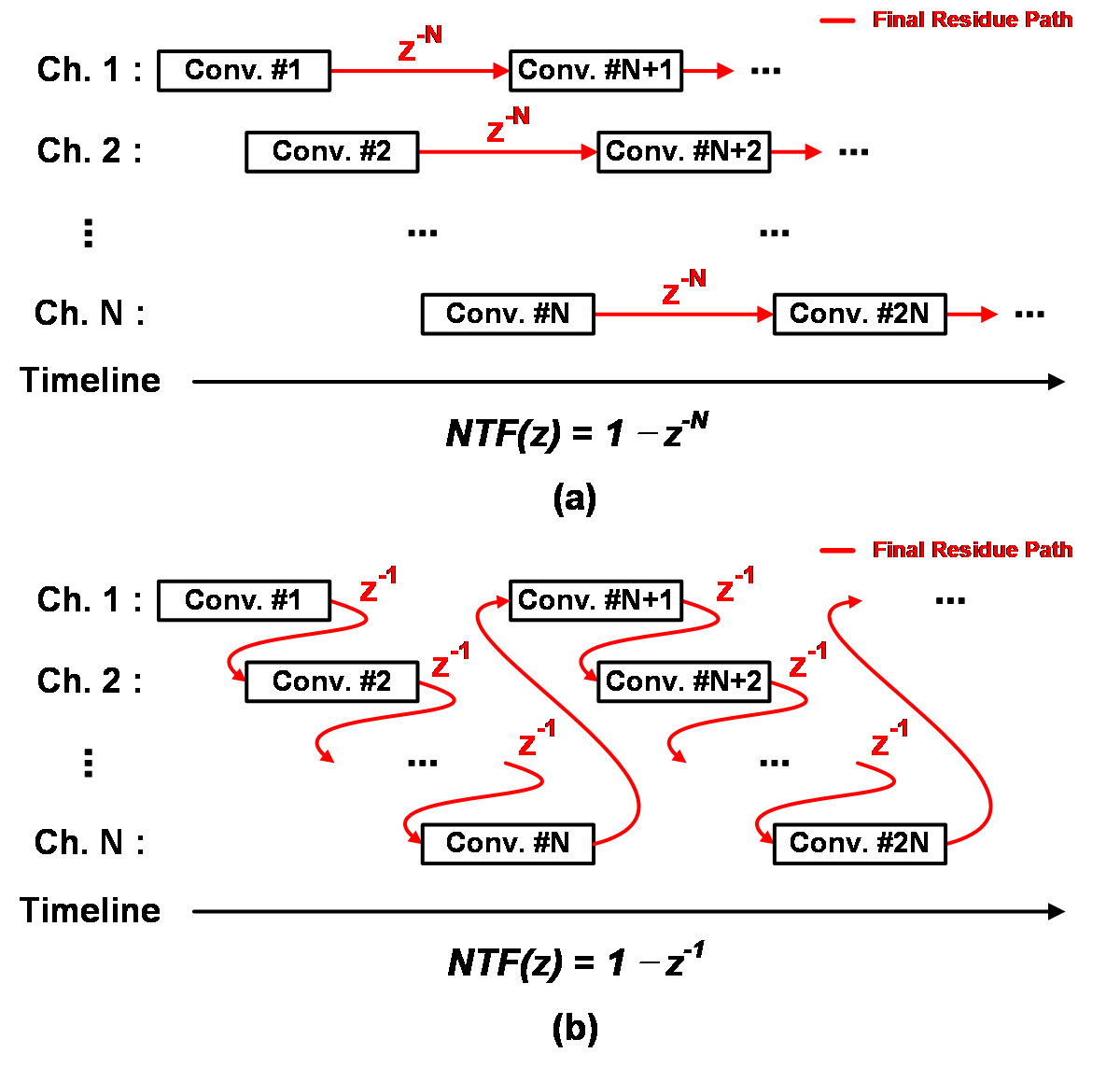Fig. 2. Midway feedback for TINS-SAR ADC using inter-channel feedback.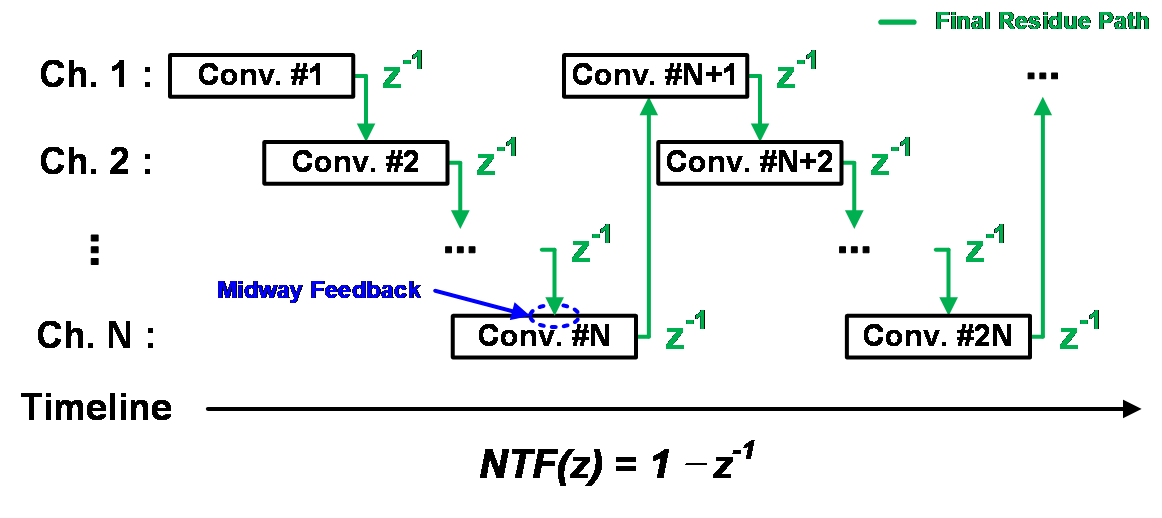The alternative final residue feedback method, inter-channel feedback, involves feeding the final residue generated by current channel to the next channel. This approach has only one-sample feedback delay (z$^{-1}$), as shown in Fig. 1(b), and thus, an NTF of (1-z$^{-1}$) can be implemented with a single in-band notch. However, a timing problem occurs, as the final residues are not generated by the current channel before the conversion of the next channel is initiated. The addition of an artificial delay to solve the timing problem is not desirable because the overall conversion speed is significantly reduced.

In the prior work (5), the timing problem is solved using midway feedback, by passing the final residue generated by the current channel to the next channel in the middle of the conversion of the next channel, as shown in Fig. 2. Because the SAR conversion process consists of several repetitions of comparison cycles, it is easy to feedback the final residue before a certain comparison cycle. In addition, because the quantization noise of all conversion cycles except the last conversion is cancelled, the TINS-SAR ADC with midway feedback can obtain the same NS effect as that of the conventional NS-SAR ADC, thereby avoiding any overhead of resolution and speed.

Fig. 3. NS-SAR ADC architecture based on (a) EF, (b) CIFF.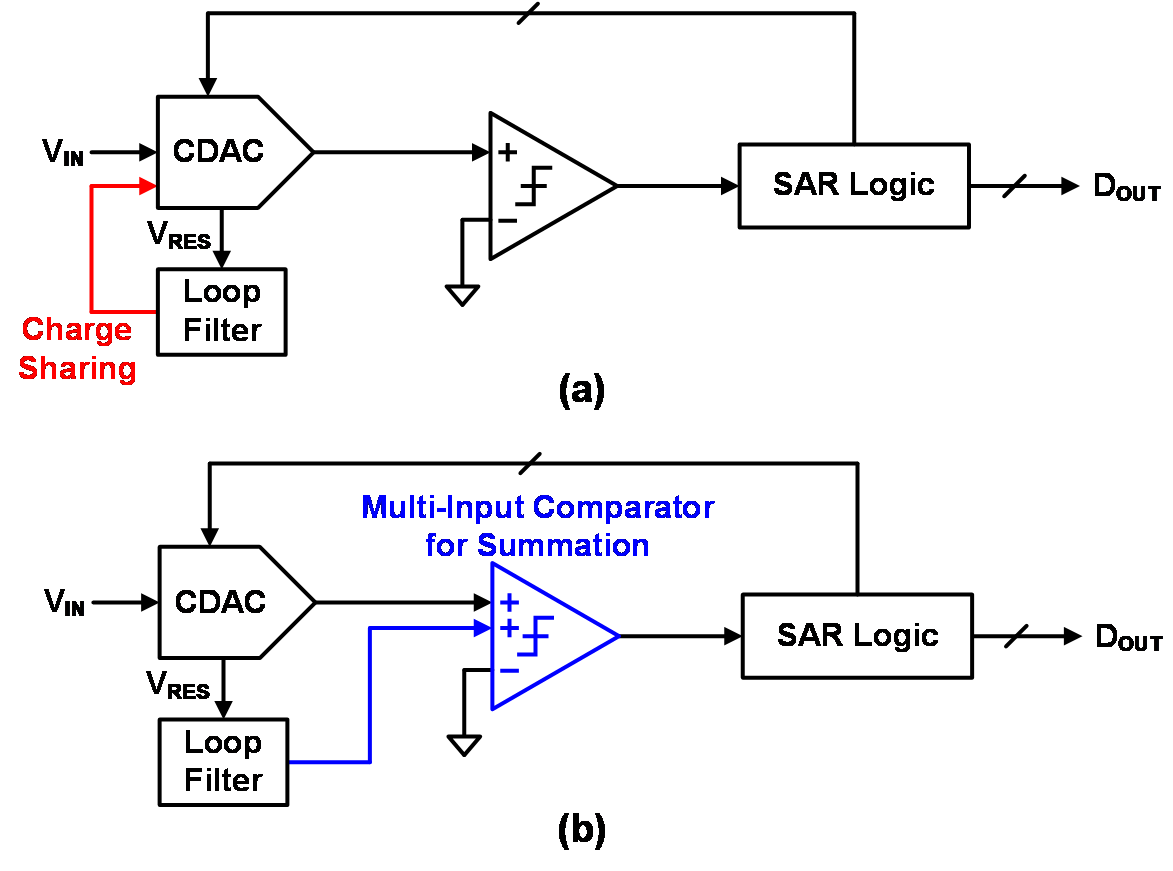### 2. TINS-SAR ADC based on CIFF Architecture

The two main architectures used to implement a NS-SAR ADC are the EF and CIFF architectures (6). Fig. 3(a) shows the NS-SAR ADC based on the EF architecture. This system feeds the final residue back to the CDAC and performs the summation of the CDAC voltage and final residue through charge sharing process. In this case, when the aforementioned midway feedback is used, the signal sampled to the CDAC is attenuated by charge sharing, owing to which, the quantization error is not cancelled in the next conversion. In the prior work (5), the CDAC voltage and final residue is summed using the summation pre-amplifier to solve this problem. However, this pre-amplifier consumes static power, reduces the overall speed, and increases the overall circuit complexity because it is necessary for all channels.

To overcome the limitations of using the summation pre-amplifier, the proposed ADC uses the CIFF architecture shown in Fig. 3(b). Unlike the EF architecture, the CIFF architecture uses a dynamic multi-input comparator to sum the CDAC voltage and final residue. Therefore, this architecture does not require a summation pre-amplifier because the CDAC voltage remains the same regardless of the midway feedback.

Fig. 4. (a) Signal flow, (b) block diagram of the proposed ADC.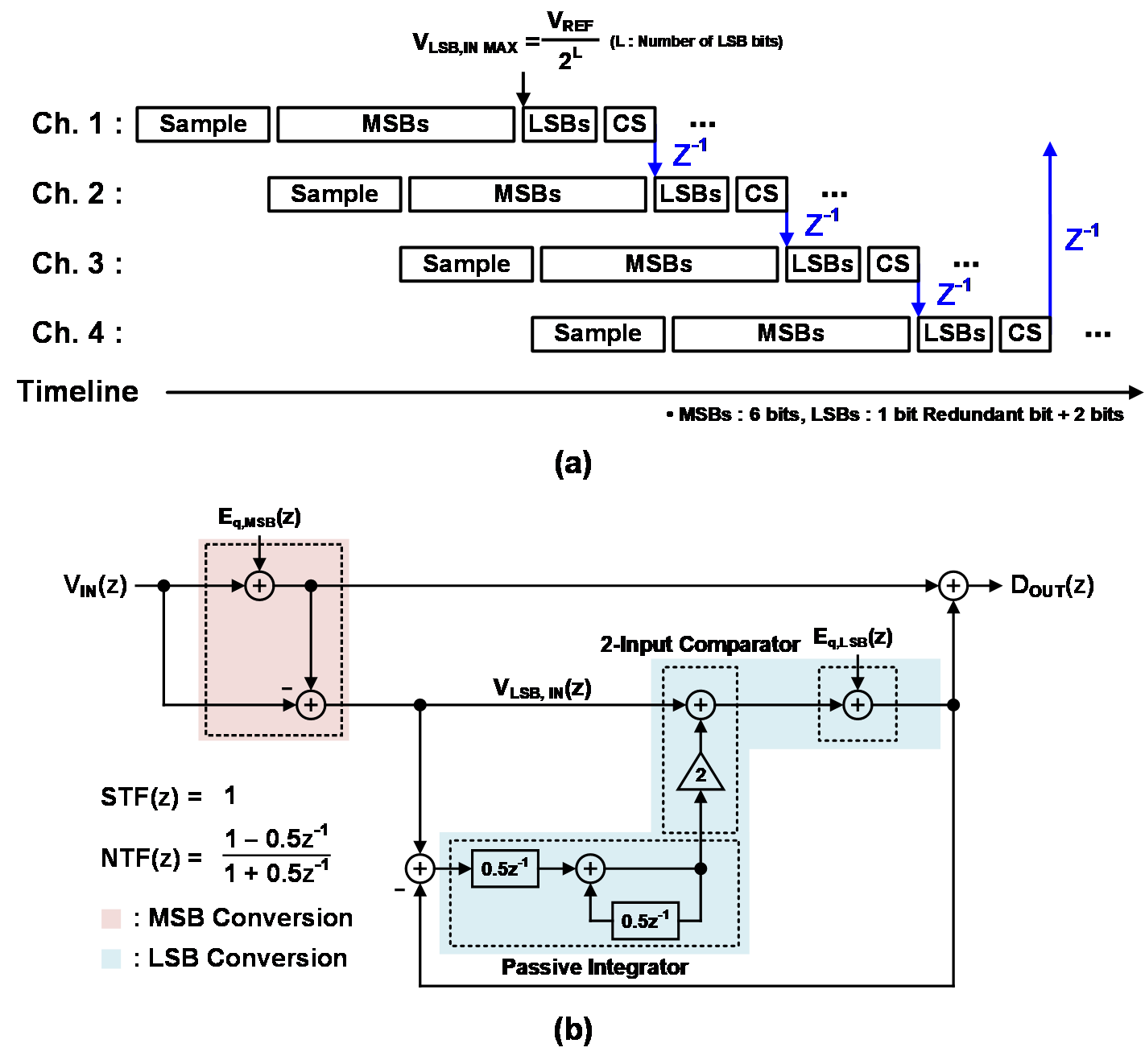Fig. 4(a) shows the signal flow of the proposed four-channel 10-bit TINS-SAR ADC based on the CIFF architecture. Each channel performs 8-bit most significant bit (MSB) conversions followed by the least significant bit (LSB) conversions including the final residue generated and passed from the previous channel. The proposed ADC, as shown in Fig. 4(b), uses a two-input comparator to sum the CDAC voltage and final residue of the previous channel, thereby attaining a 2${\times}$ gain by adjusting the device size ratio. Therefore, the first-order NTF of (1-0.5z$^{-1}$)/(1+0.5z$^{-1}$) can be implemented without an amplifier by using a passive integrator based on a switched capacitor (SC) (7). When the LSB conversion is initiated, one redundant bit (1-bit) is added, which prevents the overflow caused by adding a final residue in the middle of the conversion. The redundancy provides an additional LSB conversion range to solve the problem of exceeding the maximum LSB conversion range (V$_{\mathrm{LSB, IN MAX}}$) (8). After the redundant bit, 2-bit LSB conversions are performed to complete the analog-to-digital conversion.

## III. CIRCUIT IMPLEMENTATION

Fig. 5 shows the top-level circuit implementation of the proposed ADC. The proposed ADC consists of four channels, and the final output can be obtained by combining the results of each channel through a multiplexer (MUX). In the prior work (5), a summation pre-amplifier and final residue sampling capacitor (C$_{\mathrm{RES}}$) is used for every channel. Since each channel also adds a switch for sampling residues of other channels and logic to control it, this increases the complexity of the circuit. In contrast, in the proposed ADC, all the channels share only one C$_{\mathrm{RES}}$ to sample the final residue. The power consumption and circuit complexity are further reduced because pre-amplifiers and additional logic are not required, as well as simplifying metal routing between channels. At this time, the size of C$_{\mathrm{RES }}$is 514C (C = 2 fF), same to that of the CDAC.

The 10-bit CDAC uses the merged capacitor switching (MCS) scheme (9). Notably, the MCS can maintain a common-mode voltage with a high switching efficiency, and it can implement the same operation in half the size compared to conventional switching. In addition, the CDAC includes a 4-LSB magnitude redundancy (2C) to provide the aforementioned additional LSB conversion range.

The comparator used in the proposed ADC uses a double-tail dynamic comparator to reduce the kickback noise (10). In the proposed ADC, a multi-input comparator is used to serve two functions: summation and amplification. Additional input pairs are used to sum the CDAC voltage and final residue. In this case, as shown in Fig. 5, the devices connected to the RES node and INT node are set as 1:2 to obtain a 2${\times}$ gain, which eliminates the need for an additional amplifier.

The proposed ADC uses asynchronous logic to ensure a high conversion speed (11,12). Fig. 6 shows the operation of each channel. First, input signal sampling is performed through a bootstrapped switch (13), and then 8-bit MSB conversions are performed. At this time, the INT node is reset to V$_{\mathrm{CM}}$. After the MSB conversions, C$_{\mathrm{RES}}$, which contains the final residue generated in the previous channel, is connected to the RES node as $\overline{\Phi }$ goes low and $\Phi$ goes high (the two clocks are non-overlapped), and LSB conversions are performed.

## IV. POST-LAYOUT SIMULATION RESULTS

This section discusses the post-layout simulation results of the proposed TINS-SAR ADC based on the CIFF architecture. The circuit is implemented in a 65-nm CMOS process. As shown in Fig. 7, the size of the ADC core is 360 ${\mathrm{\mu}}$m ${\times}$ 250 ${\mathrm{\mu}}$m, and the single channel occupies an area of 165 ${\mathrm{\mu}}$m ${\times}$ 105 ${\mathrm{\mu}}$m. The post-layout simulation contains transient noise up to 100 GHz. Fig. 8 shows the output spectral density of the proposed ADC at a sampling rate of 800 MHz. The input signal has magnitude of -0.82 dBFS at 8.2 MHz. The results show that the SNDR of 69.2 dB is obtained at a bandwidth of 100 MHz (OSR = 4).

Fig. 8. Output power spectral density of the proposed ADC from post-layout simulation.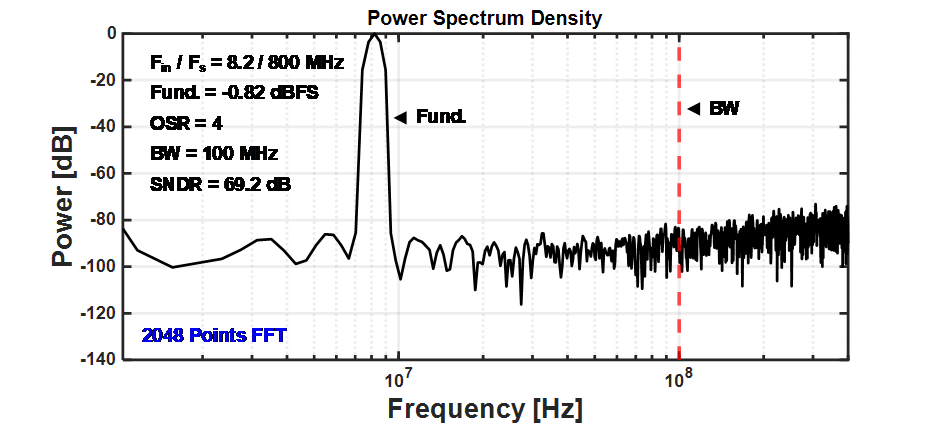The total power consumption is 8.6 mW under a supply voltage of 1.2 V. The proportions of digital, analog, and CDAC are 5 mW, 2.5 mW, and 1.1 mW, respectively. As shown in Fig. 9, by replacing the pre-amplifier with the multi-input comparator, the proposed ADC significantly reduces the power consumption associated by the pre-amplifier, which is approximately 45.3% in the prior work.

Fig. 9. Pie chart of power consumption (a) prior work (based on EF), (b) proposed ADC (based on CIFF).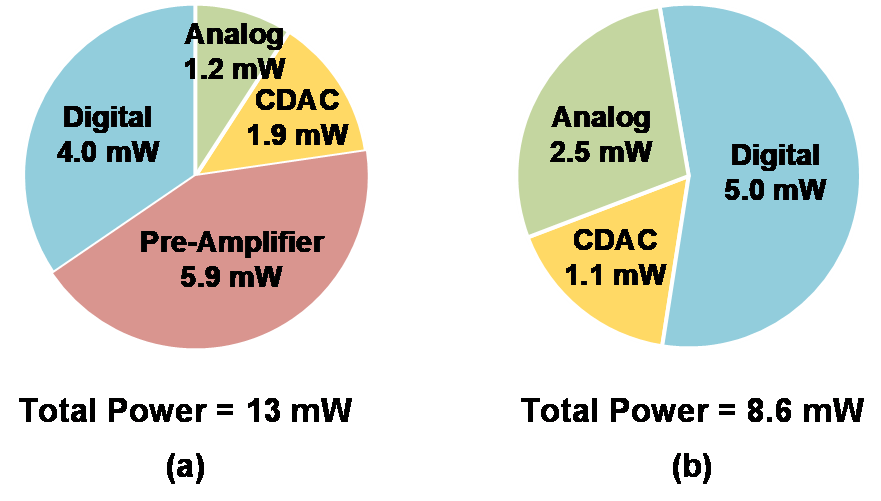Table 1. Performance and specifications of the prior works and the proposed ADC

   This Work Architecture NS-SAR TINS-SAR (EF) TINS-SAR (CIFF) Amplifier YES YES NO Process (nm) 65 40 65 Area (mm2) 0.0462 0.061 0.09 Supply Voltage (V) 1.2 1 1.2 Power (mW) 0.8 13 8.6 Sampling Rate (MS/s) 90 400 800 OSR 4 4 4 Bandwidth (MHz) 11 50 100 SNDR (dB) 62.1 70.4 69.2 FoMS (dB) 163.3 166.3 169.9 FoMw (fJ/conv.-step) 35.8 48.1 18.2

FoMs $=S N D R+10 \cdot \log 10($ Bandwidth/Power $)$

FoMw $=$ Power $\left / (2^{\text {ENOB }} \cdot 2\right.$ Bandwidth $)$.

*ENOB $=$ Effective number of bits.

Table 1 presents the performance values and specifications of the prior works and proposed ADC. The TINS-SAR ADC based on the CIFF architecture achieves Schreier figure of merit (FoM$_{\mathrm{S}}$) of 169.9 dB and Walden figure of merit (FoM$_{\mathrm{W}}$) of 18.2 fJ/conv.-step. The proposed ADC exhibits a high conversion speed with an energy efficiency comparable to that of the conventional NS-SAR ADC. Compared with a TINS-SAR ADC based on EF (5), its energy efficiency (FoMs) is 3.6 dB better because it does not use an amplifier.

## IV. CONCLUSIONS

The proposed TINS-SAR ADC based on CIFF can obtain a high resolution with a high energy efficiency, by exploiting the advantages of the SAR ADC and using NS and oversampling. Moreover, the proposed ADC can expand the limited bandwidth by increasing the sampling rate through interleaving. In contrast to the prior TINS-SAR ADC based on EF, the power consumption is reduced as an amplifier that consumes static power is not used. The circuit complexity is also reduced because all channels share only one residue sampling capacitor.

### ACKNOWLEDGMENTS

This work was supported by Konkuk University in 2019.

### REFERENCES

1
Son W.-S., Seo Y. K., Kim W.-J., Kim H.-N., Aug 2018, Analysis of signal transmission methods for rapid searching in active SONAR systems, J. IEIE, Vol. 55, No. 8, pp. 83-932
Lin Y., Feb. 2019, A 40MHz-BW 320MS/s Passive Noise-Shaping SAR ADC With Passive Signal-Residue Summation in 14nm, in IEEE Int. Solid-State Circuits Conf. (ISSCC) Dig. Tech. Papers, San Francisco, CA, USA, pp. 330-3323
Zhuang. H., June 2019, A Second-Order Noise-Shaping SAR ADC With Passive Integrator and Tri-Level Voting, IEEE J. Solid-State Circuits, Vol. 54, No. 6, pp. 1636-16474
Liu. J., Feb. 2020, A 40kHz-BW 90dB-SNDR Noise-Shaping SAR with 4× Passive Gain and 2nd-Order Mismatch Error,, San Francisco, Vol. ca, No. usa, pp. 158-1605
Jie L., Zheng. B., Flynn M. P., Dec 2019, A Calibration-Free Time-Interleaved Fourth-Order Noise-Shaping SAR ADC, IEEE J. Solid-State Circuits, Vol. 54, No. 12, pp. 3386-33956
Salgado G. M., O’Hare D., O’Connell I., Exp Briefs, Recent advances and trends in noise shaping SAR ADCs, IEEE Trans. Circuits Syst. II, Vol. 68, No. 2, pp. 545-5497
Zhuang. H., Jun 2019, A second-order noise-shaping SAR ADC with passive integrator and tri-level voting, IEEE J. Solid-State Circuits, Vol. 54, No. 6, pp. 1636-16478
Hesener. M., Feb. 2007, A 14b 40 MS/s Redundant SAR ADC with 480 MHz Clock in 0.13 pm CMOS, in IEEE Int. Solid-State Circuits Conf. (ISSCC) Dig. Tech. Papers, San Francisco, CA, USA, pp. 248-2499
Hariprasath. V., April 2010, Merged capacitor switching based SAR ADC with highest switching energy-efficiency, Electronics Letters, Vol. 46, pp. 620-62110
Shinkel. D., Feb. 2007, A double-tail latch-type voltage sense amplifier with 18ps Setup+Hold time, in Proc. IEEE Int. Solid-State Circuits Conf., Vol. dig. tech. papers, pp. 314-31511
Chen S.-W. M., Brodersen R. W., Dec 2006, A 6-bit 600-MS/s 5.3-mW asynchronous ADC in 0.13 CMOS, IEEE J. Solid-State Circuits, Vol. 41, No. 12, pp. 2669-268012
Liu. C.-C., Apr 2010, A 10-bit 50-MS/s SAR ADC with a monotonic capacitor switching procedure, IEEE J. Solid-State Circuits, Vol. 45, No. 4, pp. 731-74013
Abo A. M., Gray P. R., May 1999, A 1.5-V, 10-bit, 14.3-MS/s CMOS pipeline analog-to-digital converter, IEEE J. Solid-State Circuits, Vol. 34, No. 5, pp. 599-606## Author

Ki-Hyun Kim received the B.S. degrees in electrical engineering from Kookmin University, Seoul, Korea, in 2020.

He is currently working toward the M.S. degree in electrical engineering at Konkuk University.

His research interests include data converter.

Ji-Hyun Baek received the B.S. degree in electrical engineering from Konkuk University, Seoul, Korea, in 2021.

She is currently working toward the M.S. degree in electrical engineering at Konkuk University.

Her research during the M.S. course has focused on high speed ADC.

Jong-Hyun Kim is currently undergraduate student in electrical engineering from Konkuk University, Seoul, Korea.

His research has focused on analog to digital converter and digital calibration.

Hyung-Il Chae received the B.S. degree in electrical engineering from Seoul National University, Seoul, Korea, in 2004, and his M.S. and Ph.D. degrees in electrical engi-neering from the University of Michigan, Ann Arbor, MI in 2009 and 2013, respectively.

From 2013 to 2015, he was a senior engineer at Qualcomm Atheros, San Jose, CA.